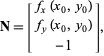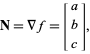### Introduction

The normal vector, often simply called the "normal", to a surface is a vector perpendicular to it. Often, the normal unit vector is desired, which is sometimes known as the "unit normal".

The normal vector at point (x0, y0) on a surface z = f(x ,y) is given bywhereandare partial derivatives.

A normal vector to a plane is specified byis given bywheredenotes the gradient.

### References & Resources

• http://mathworld.wolfram.com/NormalVector.html